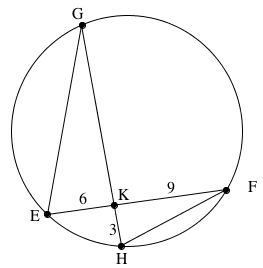### Home > INT2 > Chapter 10 > Lesson 10.2.4 > Problem10-96

10-96.

Review circle relationships as you answer the questions below.

1. On your paper, draw a diagram of $⊙B$ with $\overarc { A C }$. If $m\overarc { A C }= 80°$ and the length of the radius of $⊙B$ is $10$, what is the length of chord $\overline{AC}$?

What do you notice about $BA$ and $BC$?

How can trigonometry help you solve for $AC$?

2. Now draw a diagram of a circle with two chords, $\overline{EF}$ and $\overline{GH}$, that intersect at point $K$. If $EF = 15$, $EK = 6$, and $HK = 3$, what is $GK$?Remember that intersecting chords create similar
triangles, and that the corresponding sides of similar
triangles have equal ratios.

$\frac{9}{3}=\frac{x}{6}$